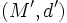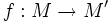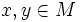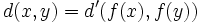# Difference between revisions of "Isometry"

## Definition

### Symbol-free definition

A function from a metric space (often, a Riemannian manifold) to another is termed an isometry if it preserves distance between points.

### Definition with symbols

Let$(M,d)$ and$(M',d')$ be metric spaces. A function$f: M \to M'$ is termed an isometry if for all$x,y \in M$:$d(x,y) = d'(f(x),f(y))$

We are often concerned with self-isometries of a metric space, viz isometries from a metric space to itself.